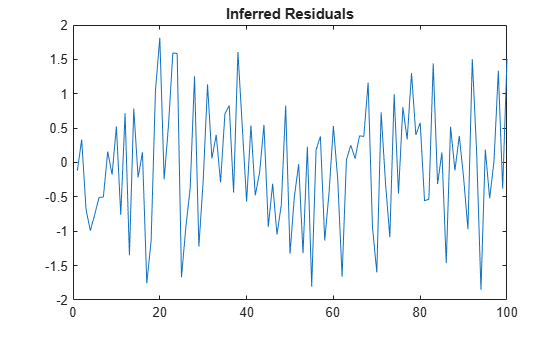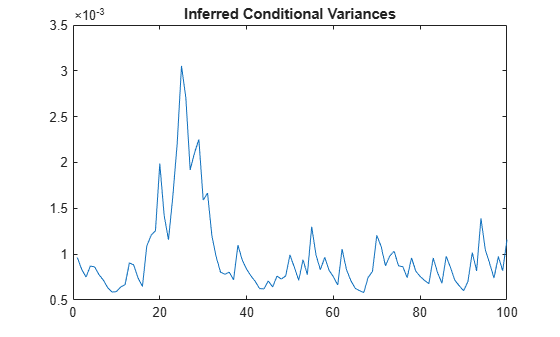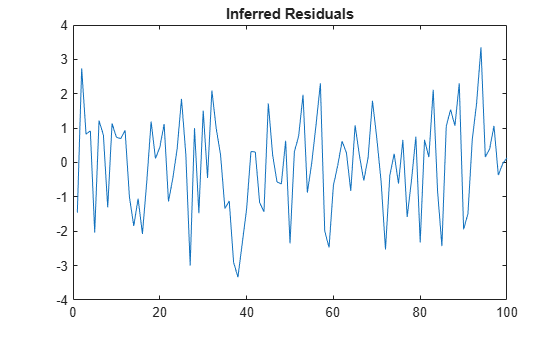# infer

Infer ARIMA or ARIMAX model residuals or conditional variances

## Syntax

```[E,V] = infer(Mdl,Y) [E,V,logL] = infer(Mdl,Y) [E,V,logL] = infer(Mdl,Y,Name,Value) ```

## Description

```[E,V] = infer(Mdl,Y)``` infers residuals and conditional variances of a univariate ARIMA model fit to data `Y`.

```[E,V,logL] = infer(Mdl,Y)``` additionally returns the loglikelihood objective function values.

`[E,V,logL] = infer(Mdl,Y,Name,Value)` infers the ARIMA or ARIMAX model residuals and conditional variances, and returns the loglikelihood objective function values, with additional options specified by one or more `Name,Value` pair arguments.

## Input Arguments

expand all

Fully specified ARIMA model, specified as an `arima` model object created by `arima` or `estimate`.

The properties of `Mdl` cannot contain `NaN` values.

Response data, specified as a numeric column vector or numeric matrix. If `Y` is a matrix, then it has `numObs` observations and `numPaths` separate, independent paths.

`infer` infers the residuals and variances of `Y`. `Y` represents the time series characterized by `Mdl`, and it is the continuation of the presample series `Y0`.

• If `Y` is a column vector, then it represents one path of the underlying series.

• If `Y` is a matrix, then it represents `numObs` observations of `numPaths` paths of an underlying time series.

`infer` assumes that observations across any row occur simultaneously. The last observation of any series is the latest.

Data Types: `double`

### Name-Value Arguments

Specify optional pairs of arguments as `Name1=Value1,...,NameN=ValueN`, where `Name` is the argument name and `Value` is the corresponding value. Name-value arguments must appear after other arguments, but the order of the pairs does not matter.

Before R2021a, use commas to separate each name and value, and enclose `Name` in quotes.

Presample innovations that have mean 0 and provide initial values for the model, specified as the comma-separated pair consisting of `'E0'` and a numeric column vector or numeric matrix.

`E0` must contain at least `numPaths` columns and enough rows to initialize the ARIMA model and any conditional variance model. That is, `E0` must contain at least `Mdl.Q` innovations, but can be greater if you use a conditional variance model. If the number of rows in `E0` exceeds the number necessary, then `infer` only uses the latest observations. The last row contains the latest observation.

If the number of columns exceeds `numPaths`, then `infer` only uses the first `numPaths` columns. If `E0` is a column vector, then `infer` applies it to each inferred path.

Data Types: `double`

Presample conditional variances providing initial values for any conditional variance model, specified as the comma-separated pair consisting of `'V0'` and a numeric column vector or matrix with positive entries.

`V0` must contain at least `numPaths` columns and enough rows to initialize the variance model. If the number of rows in `V0` exceeds the number necessary, then `infer` only uses the latest observations. The last row contains the latest observation.

If the number of columns exceeds `numPaths`, then `infer` only uses the first `numPaths` columns. If `V0` is a column vector, then `infer` applies it to each inferred path.

By default, `infer` sets the necessary observations to the unconditional variance of the conditional variance process.

Data Types: `double`

Exogenous predictor data for the regression component, specified as the comma-separated pair consisting of `'X'` and a matrix.

The columns of `X` are separate, synchronized time series, with the last row containing the latest observations.

If you do not specify `Y0`, then the number of rows of `X` must be at least `numObs + Mdl.P`. Otherwise, the number of rows of `X` should be at least `numObs`. In either case, if the number of rows of `X` exceeds the number necessary, then `infer` uses only the latest observations.

By default, the conditional mean model does not have a regression coefficient.

Data Types: `double`

Presample response data that provides initial values for the model, specified as the comma-separated pair consisting of `'Y0'` and a numeric column vector or numeric matrix. `Y0` must contain at least `Mdl.P` rows and `numPaths` columns. If the number of rows in `Y0` exceeds `Mdl.P`, then `infer` only uses the latest `Mdl.P` observations. The last row contains the latest observation. If the number of columns exceeds `numPaths`, then `infer` only uses the first `numPaths` columns. If `Y0` is a column vector, then `infer` applies it to each inferred path.

By default, `infer` backcasts to obtain the necessary observations.

Data Types: `double`

Notes

• `NaN`s indicate missing values and `infer` removes them. The software merges the presample data and main data sets separately, then uses list-wise deletion to remove any `NaN`s. That is, `infer` sets `PreSample` = `[Y0 E0 V0]` and `Data` = `[Y X]`, then it removes any row in `PreSample` or `Data` that contains at least one `NaN`.

• The removal of `NaN`s in the main data reduces the effective sample size. Such removal can also create irregular time series.

• `infer` assumes that you synchronize the response and predictor series such that the latest observation of each occurs simultaneously. The software also assumes that you synchronize the presample series similarly.

• The software applies all exogenous series in `X` to each response series in `Y`.

## Output Arguments

expand all

Inferred residuals, returned as a numeric matrix. `E` has `numObs` rows and `numPaths` columns.

Inferred conditional variances, returned as a numeric matrix. `V` has `numObs` rows and `numPaths` columns.

Loglikelihood objective function values associated with the model `Mdl`, returned as a numeric vector. `logL` has `numPaths` elements associated with the corresponding path in `Y`.

Data Types: `double`

## Examples

expand all

Infer residuals from an AR model.

Specify an AR(2) model using known parameters.

```Mdl = arima('AR',{0.5,-0.8},'Constant',0.002,... 'Variance',0.8);```

Simulate response data with 102 observations.

```rng 'default'; Y = simulate(Mdl,102);```

Use the first two responses as presample data, and infer residuals for the remaining 100 observations.

```E = infer(Mdl,Y(3:end),'Y0',Y(1:2)); figure; plot(E); title 'Inferred Residuals';```Infer the conditional variances from an AR(1) and GARCH(1,1) composite model.

Specify an AR(1) model using known parameters. Set the variance equal to a `garch` model.

```Mdl = arima('AR',{0.8,-0.3},'Constant',0); MdlVar = garch('Constant',0.0002,'GARCH',0.6,... 'ARCH',0.2); Mdl.Variance = MdlVar;```

Simulate response data with 102 observations.

```rng 'default'; Y = simulate(Mdl,102);```

Infer conditional variances for the last 100 observations without using presample data.

`[Ew,Vw] = infer(Mdl,Y(3:end));`

Infer conditional variances for the last 100 observations using the first two observations as presample data.

`[E,V] = infer(Mdl,Y(3:end),'Y0',Y(1:2));`

Plot the two sets of conditional variances for comparison. Examine the first few observations to see the slight difference between the series at the beginning.

```figure; subplot(2,1,1); plot(Vw,'r','LineWidth',2); hold on; plot(V); legend('Without Presample','With Presample'); title 'Inferred Conditional Variances'; hold off subplot(2,1,2); plot(Vw(1:5),'r','LineWidth',2); hold on; plot(V(1:5)); legend('Without Presample','With Presample'); title 'Beginning of Series'; hold off```Infer residuals from an ARMAX model.

Specify an ARMA(1,2) model using known parameters for the response (`MdlY`) and an AR(1) model for the predictor data (`MdlX`).

```MdlY = arima('AR',0.2,'MA',{-0.1,0.6},'Constant',... 1,'Variance',2,'Beta',3); MdlX = arima('AR',0.3,'Constant',0,'Variance',1);```

Simulate response and predictor data with 102 observations.

```rng 'default'; % For reproducibility X = simulate(MdlX,102); Y = simulate(MdlY,102,'X',X);```

Use the first two responses as presample data, and infer residuals for the remaining 100 observations.

```E = infer(MdlY,Y(3:end),'Y0',Y(1:2),'X',X); figure; plot(E); title 'Inferred Residuals'; ```## References

 Box, G. E. P., G. M. Jenkins, and G. C. Reinsel. Time Series Analysis: Forecasting and Control 3rd ed. Englewood Cliffs, NJ: Prentice Hall, 1994.

 Enders, W. Applied Econometric Time Series. Hoboken, NJ: John Wiley & Sons, 1995.

 Hamilton, J. D. Time Series Analysis. Princeton, NJ: Princeton University Press, 1994.